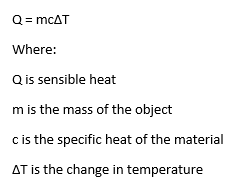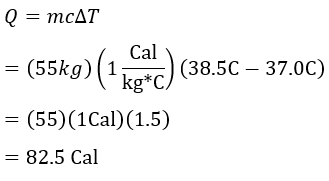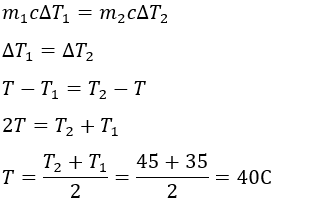# Sensible Heat: Definition, Load & Equation

Instructor
John Parsons

John has taught physics to both engineers and non-engineers, and has a master's degree in Civil and Environmental Engineering.

Expert Contributor
Christianlly Cena

Christianlly has taught college physics and facilitated laboratory courses. He has a master's degree in Physics and is pursuing his doctorate study.

Sensible heat is the amount of thermal energy that is required to change the temperature of an object. This lesson will look at how sensible heat changes that temperature and the equation that we use to model the temperature change. Updated: 07/07/2021

## Changing Temperature

Heat is useful to changing the temperature of an object, whether raising or lowering the temperature. For example, when you boil a pot of water on the stove, the heat is transferred from the stove to the pot, and the water's temperature rises. Turning off the stove or taking the pot of water off the heat will cause the water to cool, lowering its temperature.

Let's take a look at how the transfer of heat will affect an object's temperature.

## Newton's Law of Heating/Cooling

First, we need to look at Newton's Law of Heating/Cooling. This tells us how thermal energy, the energy associated with heat, is transferred from one source to another. Thermal energy always moves from a warmer source to a cooler source, and this transfer will continue until the two objects are at the same temperature, or equilibrium temperature.

Looking back at our pot of water, when it is placed on a hot stove, the stove's hot burner acts as the heat source and sends thermal energy into the pot of water. This energy then excites the water molecules and causes them to move at a faster rate, increasing the water's temperature.

When the pot is taken off the heat, thermal energy is still transferring from a hot source to a cool source. In this case, the hot source is the pot of water, and the cool source is the environment. So, the hot water is trying to warm the environment around it, but because the environment is so much larger than the pot of water, it will take far more thermal energy to raise the surrounding temperature than the pot of water can give up. This causes us to notice the drop in temperature of the water, and not the increase in temperature of the environment.

## Sensible Heat

So, how does this relate to sensible heat? Sensible heat is the amount of thermal energy that is required to raise an object's temperature. It is a function of how much material there is and the type of material being heated. The amount of sensible heat required is calculated using the following equation:Sensible heat is just the energy associated with temperature change. It will not change the object's phase - whether it is a solid, liquid, or a gas - and will not change the object's pressure or volume. While it is true that increasing the temperature causes the molecules to spread out, the increase caused by sensible heat alone is not significant enough to affect the equation.

## Specific Heat

Different types of material require different amounts of sensible heat to experience the same amount of temperature change. This is because the material an object is made of will affect how easily it will either absorb or give off thermal energy. The rate at which this transfer happens is called specific heat. Specific heat is the amount of energy required to raise the temperature of a unit mass of an object one degree of temperature. In other words, it is the amount of energy required to raise the temperature of an object that has a mass of one kilogram by one degree Celsius.

To unlock this lesson you must be a Study.com Member.

## Sensible Heat: Multiple Choice Exercise

### Multiple Choice

1.) In the equation for sensible heat, the quantity c refers to __________.

A. change in temperature

B. cooling coefficient

C. equilibrium temperature

D. specific heat of the material

2.) Jake has a fever of 38.5 C (where C stands for Celsius). If he weighs 55 kilograms, how much heat was required to raise his temperature to that point? Set 37 C as the normal body temperature. Lastly, assume that the body is made mostly of water with specific heat equal to 1 Cal/(kg*C).

A. 83.0 Cal

B. 82.5 Cal

C. 81.5 Cal

D. Not enough information given

3.) Which of the following statements is TRUE about sensible heat?

A. The smaller the mass of an object, the bigger the thermal energy required to raise its temperature.

B. An object's pressure or volume may change depending on the value of sensible heat.

C. Sensible heat is directly proportional to the change in temperature.

D. Both A and C

4.) Suppose you combine 20 grams of 35 C water and 50 grams of 45 C water in a container. What would be the final temperature of the mixture? (Hint: The heat lost by the warmer water is equal to the heat gained by the cooler water.)

A. 40 C

B. 100 C

C. 20 C

D. 80 C

5.) The rate at which a material absorbs or gives off thermal energy is known as specific heat.

A. TRUE

B. FALSE

1.) D

2.) B3.) C

4.) A5.) A

### Register to view this lesson

Are you a student or a teacher?